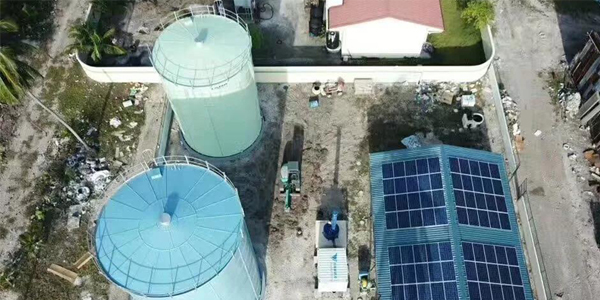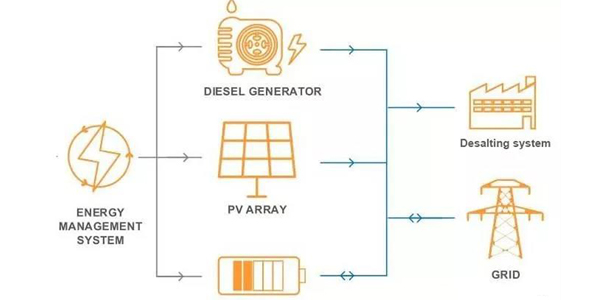# How to Calculate Solar System Size?

Oct 21, 2021

Sizing your PV system to your electricity bill, the electricity tariff usually reveals information about the total monthly energy consumption of a residential or commercial customer. From this value alone, it is possible to estimate the PV system size required to offset the monthly energy usage.

Assume a monthly energy consumption of 500 kWh, which is at the low end of the scale for a household in California. Assuming 30 days in a month, the average daily energy use-value is obtained by dividing the monthly use by 30.

Daily energy use = monthly energy use / number of days per month

= 500 k W h / m o / 30 d a y s / m o = 16.7 k W h / d a y

Next, the insolation values are required.  Insolation values are reported in kWh/m 2 - days. Since the "full insolation" value of incident solar energy is approximated as 1 kW/m 2, the insolation value reported in kWh/m 2 days approximates the full insolation time received by a location during the day.PV System

For a Palo Alto home, the average daily irradiance value is 5.2 kWh/m 2 -days. The required power output of the PV system is calculated by dividing the daily energy use by the number of sun-filled hours per day.

Power Output=Daily Energy Use * Daily hours of full sun

=16.7kWh/day * 5.2hours/day=3.21kW

If we had a system efficiency of 100%, this would be the photovoltaic system sizing required. However, this is not the case as all PV systems have a corresponding derating factor that takes into account inefficiencies in the overall system such as panel contamination and poor electrical connections.

According to the National Renewable Energy Laboratory's PVWatts calculator, a typical derating factor is 0.84. For calculation purposes, we assume a derating factor of 80%, i.e. 0.8. To determine the photovoltaic systems sizing, the required power output is divided by the derating factor.

PV System Size=Power Output / Derate Factor

=3.21kW / 0.8=4.01kW

Based on this analysis, the approximate PV system size required to fully offset the average monthly energy use of a 500 kWh/month household in Palo Alto is approximately 4 kW.Zenergy System

## Comparing PV sizing estimates with simulation results

As this is a rough estimate, how does it compare to the actual combined design of a household with the same characteristics? Using the same conditions as above, the PV system design software found the required system size to be 4 kW, which is almost the same answer as the above estimate.

Although the answer is very close, it is important to note that this may not always be the case. For example, the power output may be significantly lower when there is a shadow on the panel. Although the shading term is included in the calculation of the derating factor, it may not accurately capture the effect of shading on the power output of the PV system. As a result, when modeling locations with shadows, the expected results are less close.

Zaiming's Hybrid Grid Solution Features 100 kw PCS, 26 kwp PV power modules, and 96 kwh storage batteries for each island. It provides about 120 kw of photovoltaic power in sunny weather and has a storage capacity that allows the system to run for nearly an hour. The local energy management system coordinates the operation mode of each power supply dynamically and reports the data to the centralized management platform.

Zenergy focuses on micro-gird solutions and fully integrated renewable energy for industry, resorts, islands, and no electricity area to significantly reduce the energy cost and carbon footprint of our clients. If you want to know more information about zenergy systems, welcome to contact us.

Tel: +86 10 6127 2381 Fax: +86 10 6021 5824 E-mail: info@zenergytech.com

Lastest Article

• Dec. 28, 2021

• Dec. 15, 2021

• Nov. 23, 2021